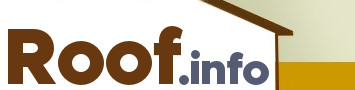• Shingle Brands

# How to Measure a Roof

There comes a time in almost every homeowner's life when repairing or replacing a roof becomes necessary. It's a big job, on even the smallest of homes, but if taken in small steps, any roof project can become manageable. The first thing that a homeowner has to do is measure the roof.

### Surface Area

Roof surfaces are measured in squares of 100 square feet each. For example, a 30,000 square foot roof equals 300 squares (30,000/100=300). When buying shingles, you would need 300 squares to cover a 30,000 square foot roof.

### The Pitch of a Roof

There are two parts of a roof pitch: the rise and the run. The run is a measure of horizontal space; in roofs, the standard run is 12 inches. The rise is a vertical measurement: how much the roof rises over 12 inches. A roof's pitch, also called the slope, is expressed as the rise over the run.

To measure the pitch, place a ladder next to - not in front of - the roof of the house. Mark out a 12 inch run by placing an L-shaped framing square against the edge of the roof so the bottom of the ruler meets the roof at the 12 inch mark. You will have created right triangle with the ruler and the roof where the roof edge is the longest side. Where the short side of the triangle meets the roof, you have marked the rise. For example, if the measuement on the short side is 5 inches, the pitch of the roof at that spot is 5/12. The pitch of a steep roof will measure 12/12; a moderately sloped roof is 6/12, and a roof whose pitch is less than 3/12 is considered flat.

Measuring a flat roof is a more clear-cut process. You can simply calculate the total square footage by multiplying the length by the width. For an accurate measurement, you will need to determine the square footage and the pitch of each separate section of roof.